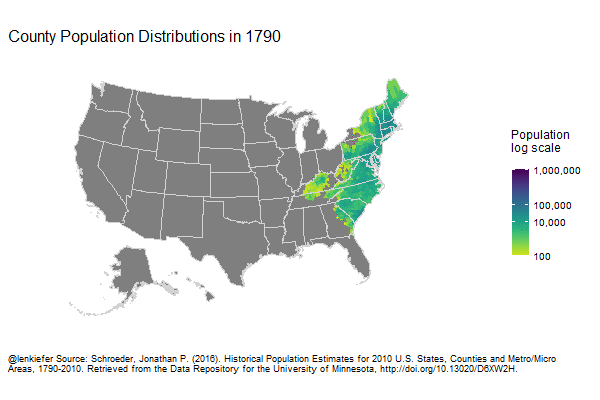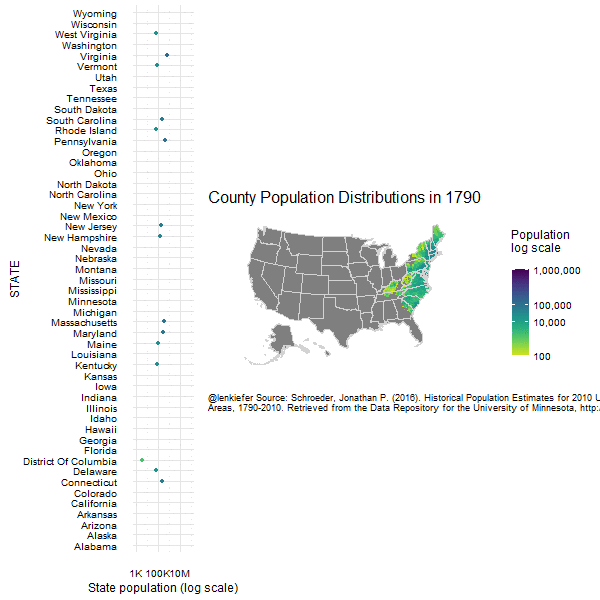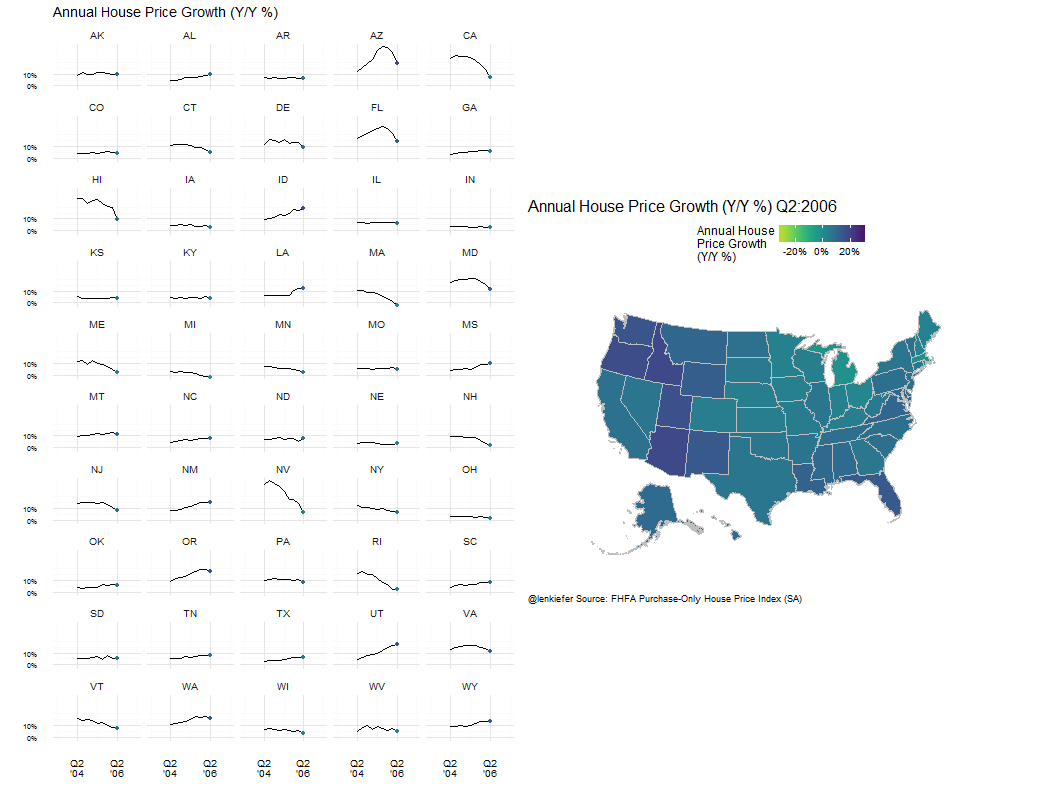More map visualizations

IN THIS POST I’M JUST GOING TO share a few data visualizations I’ve been working on today. For most, no code, but these build off my previous posts here and here so you can check there for hints and I’ll post some more examples with code later.

Population maps

This one shows the evolution of population by county without the distribution plots I included last time. We discussed these data in our last post.In this second post I combined the population map with a dot chart showing the evolution of state population.House price charts

This chart combines a map with sparkline-type line charts showing the evolution of annual percentage changes in the FHFA Purchase-Only House Price Index.Because the house price data is conveniently available in a text file from the FHFA webpage we can easily construct a static version of this chart (with just the map) using the code below.

{% highlight r #load some libraries library(data.table) library(reshape2) library(stringr) library(ggplot2) library(scales) library(ggthemes) library(rgeos) library(maptools) library(albersusa) library(dplyr) library(tidyr) library(ggalt) library(viridis) library(zoo)

#read in data available as a text file from the FHFA website: fhfa.data<-fread(“http://www.fhfa.gov/DataTools/Downloads/Documents/HPI/HPI_PO_state.txt") #create annual house price growth in the SA index: fhfa.data[,hpa12:=index_sa/shift(index_sa,4)-1,by=state] fhfa.data[,iso_3166_2:=state] #rename state to match usa_composite fhfa.data[,date:=as.Date(ISOdate(yr,qtr*3,1))] #make a date (don’t need it here) fhfa.data[,mylabel:=paste0(“Q”,qtr,”:“,yr)] #create date label for plot #do map stuff states<-usa_composite() smap<-fortify(states,region=“fips_state”) states@data <- left_join(states@data, fhfa.data, by = “iso_3166_2”)

#make plots: ggplot() + geom_map(data = smap, map = smap, aes(x = long, y = lat, map_id = id), color = “#2b2b2b”, size = 0.05, fill = NA) + geom_map(data =subset(states@data,yr>2004 & qtr==2), map = smap, aes( map_id = fips_state,fill=hpa12), color = “gray”, size = .25) + theme_map( base_size = 12) +facet_wrap(~mylabel,ncol=3)+ theme(plot.title=element_text( size = 16, margin=margin(b=10))) + theme(plot.subtitle=element_text(size = 14, margin=margin(b=-20))) + theme(plot.caption=element_text(size = 9, margin=margin(t=-15))) + coord_proj(us_laea_proj) + labs(title=“”,subtitle=“” ) + scale_fill_viridis(name = “”, discrete=F,option=“D”,end=0.95, direction=-1,limits=c(-0.35,0.35),label=percent)+ theme(legend.position = “top”) +theme(plot.caption=element_text(hjust=0,vjust=1,margin=margin(t=10)))+ labs(title=paste0(“Annual House Price Growth (Y/Y %)”), caption=“@lenkiefer Source: FHFA Purchase-Only House Price Index (SA)“) {% endhighlightWe use a small multiple to show how annual percentage gains in house prices have varied from the second quarter of 2005 through the latest data for the second quarter of 2016. The map part of the gif just morphs between facets of the plot above.

We can also construct a static version of the sparklines fairly easily:

{% highlight r mygraph.data<-subset(states@data,(yr>2004 & state !=“DC”))

ggplot(data=mygraph.data,aes(x=date,y=hpa12,group=state))+theme_minimal()+ geom_line()+
scale_color_viridis(name = “Annual House Price Growth (Y/Y %)”, discrete=F,option=“D”,end=0.95)+ scale_y_continuous(label=percent, breaks=c(-.2,-.1,0,.1,.2))+ theme(axis.text.y=element_text(size=6))+ theme(axis.text.x=element_text(size=6))+ geom_area(fill=viridis(5),alpha=0.5)+ #add shading to make seeing +/- Zero easier scale_x_date()+ geom_point(data=subset(mygraph.data,date==max(mygraph.data\$date)), aes(color=hpa12),alpha=0.75,size=2)+ theme(legend.position = “none”)+ facet_wrap(~state,ncol=10)+ theme(plot.caption=element_text(hjust=0))+ labs(x=“”,y=“”,title=“Annual House Price Growth (Y/Y %)”, caption=“@lenkiefer Source: FHFA Purchase-Only House Price Index (SA)“) {% endhighlight